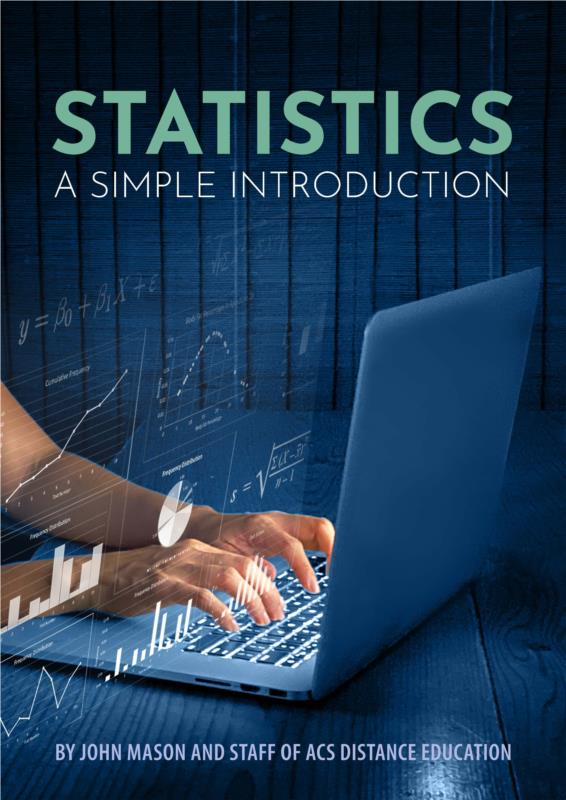# Statistics- A Simple Introduction- PDF ebook

Not Applicable

£16.95 Another currency?

 Tweet

This book was developed as a starter for anyone new to, or even struggling to come to grips with statistics.

We have been teaching statistics successfully through our online course for decades; but we have found that some people don’t need to go as deeply into the subject, and others sometimes find it challenging to undertake a course at that depth.  This ebook has been developed to be a step before that course, to provide a novice to the subject with a more digestible introduction.

CHAPTER 1 THE LANGUAGE

• Example
• Important Terms in Statistics
• Data Collection Method
• Statistical Method
• Descriptive Statistics
• Inferential Statistics
• Data Point
• Observation
• Data Set
• Research Population
• Data Sample
• Square Root
• Exponents and Roots
• Summation Sign
• Array
• Coefficient
• Constant
• Variable
• Independent and Independent Variable
• Univariate Data
• Bivariate Data
• Frequency Counts
• Frequency Distribution
• Grouped Frequency Table
• Cumulative Frequency
• Total Frequency
• Bi-Variate Frequency
• Class
• Class Interval
• Class Frequency
• Data Types
• Qualitative Data
• Quantitative Data
• Hypothesis
• Responsible Use Of Statistics

CHAPTER 2 DATA COLLECTION

• Tools for Data Collection
• Collecting Data
• Quantity
• Timing
• Time
• Contamination/Bias
• Language
• Ethics
• Cost
• Source Reliability
• Example
• Sources of Data
• Steps in Data Collection

CHAPTER 3 MEASURING THE CENTRE

• Mode, Mean, Median
• Mean
• Mode
• Median
• Range
• Standard Deviation
• Normal Distribution
• Which Measure Of Central Tendency To Use?
• Box and Whisker Plots
• Degrees Of Freedom

CHAPTER 4 DETERMINING LIKELIHOODS (INFERENTIAL STATISTICS)

• Measuring Probability
• Probability Parameters
• Discrete Variables
• Continuous Variables
• P-Values
• Statistical Significance
• Null and Alternate Hypothesis
• Errors in Hypothesis Testing
• Alpha
• Beta
• The T-Test
• Types of T-Tests
• A Review of Box and Whisker Plots Related to T-Tests
• Analysis of Variance (ANOVA)
• One-Way ANOVA
• Parametric and Non-Parametric Tests

CHAPTER 5 MEASURING CORRELATION

• Correlation
• Coefficient of Variation
• Linear Regression
• Simple Linear Regression Formula
• Conclusion
Statistics- A Simple Introduction- PDF ebook